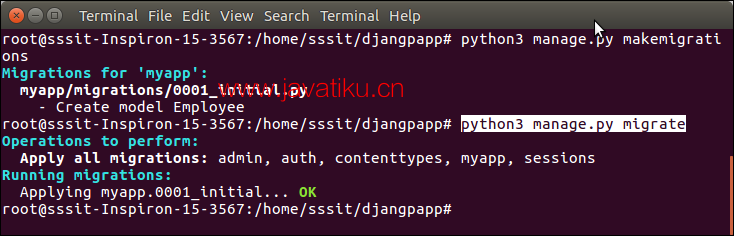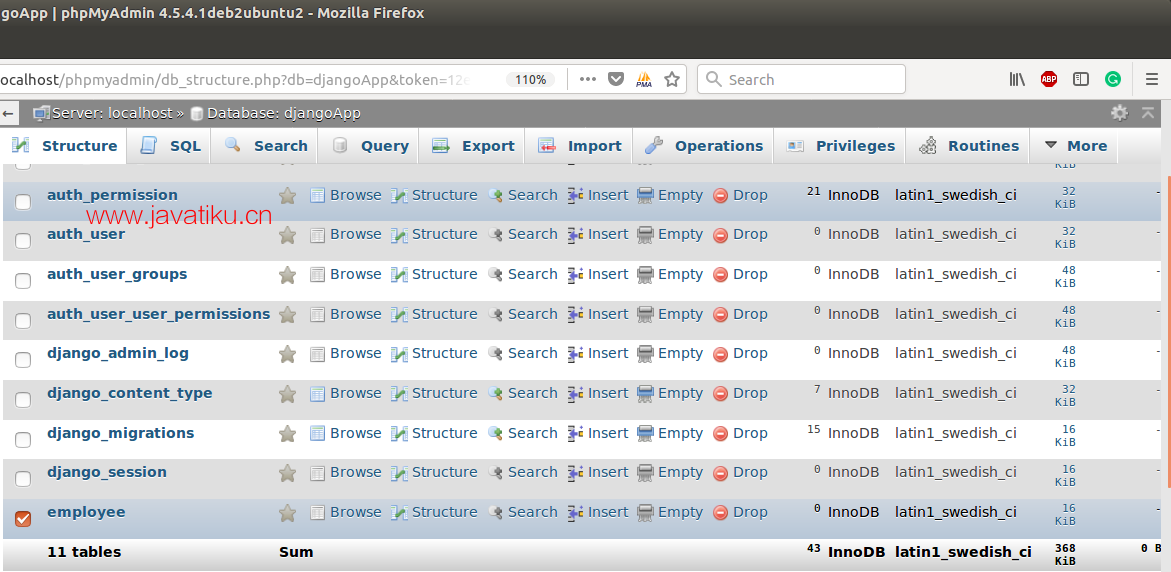# Diango教程-Django 数据库连接settings.py文件包含所有项目设置以及数据库连接详细信息。默认情况下，Django 使用SQLite数据库，也允许配置其他数据库。

``````DATABASES = {
'default': {
'ENGINE': 'django.db.backends.mysql',
'NAME': 'djangoApp',
'USER':'root',
'HOST':'localhost',
'PORT':'3306'
}
}  ``````

``\$ python3 manage.py migrate  ``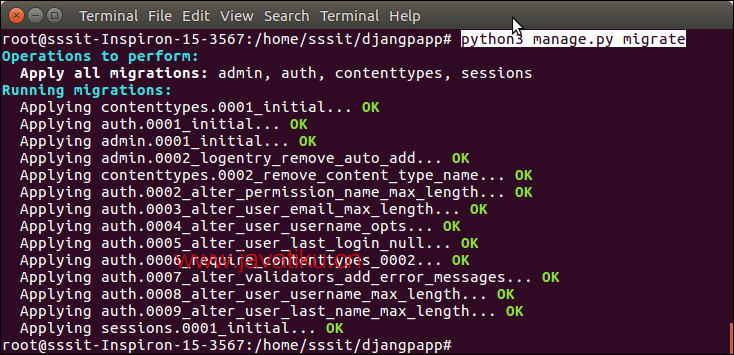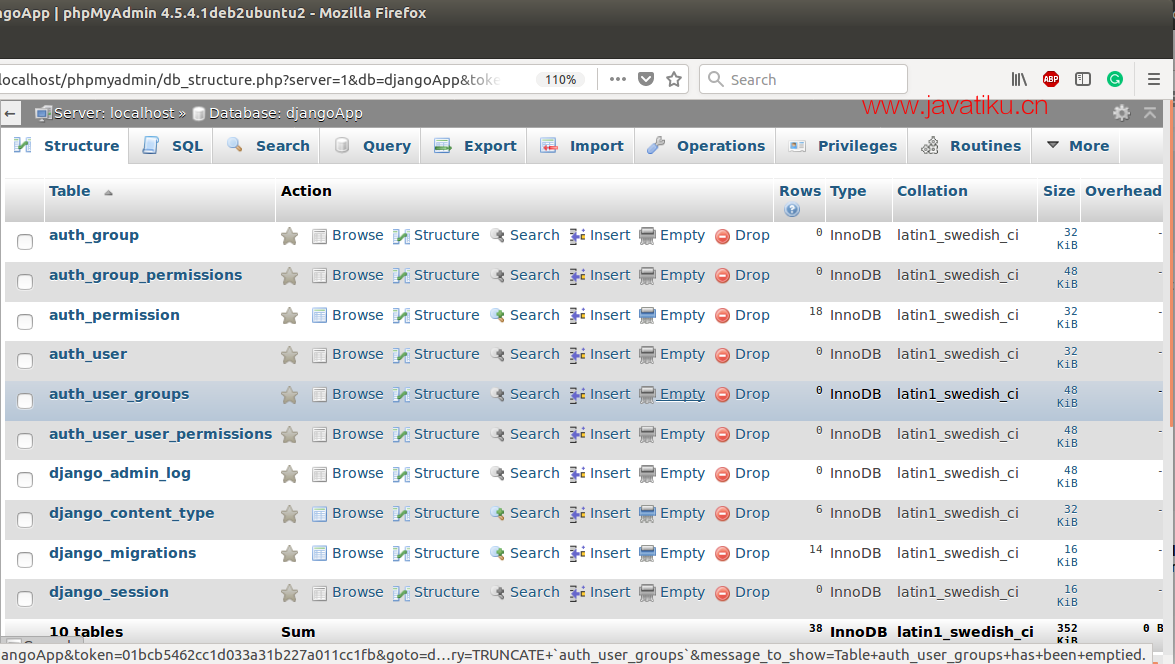## 迁移模型

// 模型.py

``````from django.db import models
class Employee(models.Model):
eid      = models.CharField(max_length=20)
ename    = models.CharField(max_length=100)
econtact = models.CharField(max_length=15)
class Meta:
db_table = "employee"  ``````

Django 首先创建一个包含表结构详细信息的迁移文件。要创建迁移，请使用以下命令。

``\$ python3 manage.py makemigrations  ``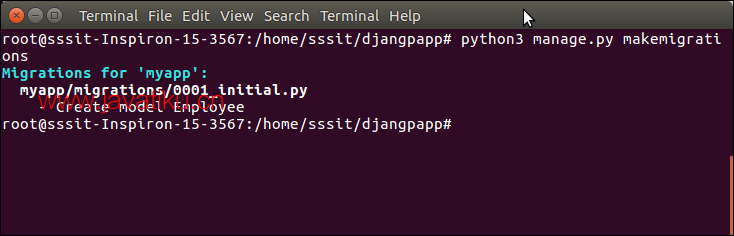``````from django.db import migrations, models
class Migration(migrations.Migration):
initial = True
dependencies = [
]
operations = [
migrations.CreateModel(
name='Employee',
fields=[
('id', models.AutoField(auto_created=True, primary_key=True, serialize=False, verbose_name='ID')),
('eid', models.CharField(max_length=20)),
('ename', models.CharField(max_length=100)),
('econtact', models.CharField(max_length=15)),
],
options={
'db_table': 'employee',
},
),
]  ``````

``\$ python3 manage.py migrate  ``